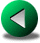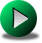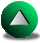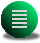Previous: Introduction
Next: The Time Discretization
Up: No Title

# Presentation of the Numerical Methods

In this chapter, we review the three numerical methods and the different models we will use in this thesis. In particular, we stress the limitations of each as it relates to the discretization of irregular domains. In the case of the spectral element method, we contribute to the development of the method by proposing our own adaptive technique. Furthermore, we present our own implementation of curved spectral elements. Although curved elements are quite natural to the spectral element method, we found very little information in the literature with respect to their implementation.

The idealized equations we propose to solve are the shallow water (SW) equations. These equations are grossly simplified compared to the primitive equations. Nonetheless, the dynamical processes involved in the formation of wind-driven circulations and the interactions with irregular coastlines are similar enough that we can restrict ourselves to these equations as an introductory study. The equations are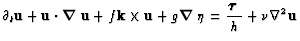(2.1)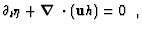(2.2)

where symbols are defined in Table 1. These equations correspond to a Boussinesq, hydrostatic, homogeneous ocean in which we assume that there is no vertical structure, reducing the real three-dimensional (3D) problem to a simple two-dimensional (2D) problem. One remark concerns the treatment of the gravity waves in these equations. The natural speed of barotropic gravity waves is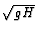where g=9.81m s-2 is the acceleration due to gravity and H is the typical oceanic depth. Since a reasonable value for H is about 4000 m, the phase speed for barotropic gravity waves is about 200 m/s. In order to use reasonable time-steps and be able to perform long time simulations, these modes have to be slowed down by using a reduced'' gravity. This approach is not inconsistent with the actual physics of the ocean. In fact, in the presence of a thermocline and a deep layer at rest below the thermocline, the SW equations with reduced gravity represent, in some sense, the first baroclinic mode dynamics, i.e., the dynamics of the upper layer. Indeed, this upper layer happens to be the location of the most intense dynamical events. The reduced gravity is defined as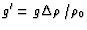where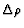is the jump in density through the pycnocline and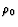the average value for the density of the ocean. For example, the Kelvin waves observed in the equatorial Pacific and along the western American coast have phase speed of 2-3 m/s [Boulanger and Fu, 1996,Ramp et al. , 1997], close to the phase speed of 3.16 m/s obtained in a SW reduced gravity model where the reduced gravity is fixed at 10-2m s-2 and the depth above the thermocline is taken to be 1000 m. Hence, these equations are consistent with a first order approximation of the physical processes involved in the layer above the thermocline.

Table 2.1: List of variables in (2.1-2.2)
 (x,y,z) the coordinate system (east-north-upward)horizontal velocity vertically averaged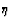elevation of the water surface taken from rest hb height of the water column above the oceanic floor at rest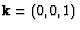unit vector normal to the horizontal plane pointing upward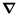gradient operator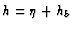fluid layer thickness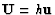vertically averaged horizontal flux of masspotential vorticity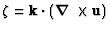relative vorticity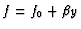Coriolis parameter varying with latitudeBernoulli function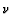dynamic eddy viscosity g'=0.01 m.s-2 reduced gravity acceleration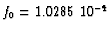s-1 defined at 450 N deg.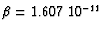m-1s-1 defined at 450 N deg. LRossby=31.22 km the Rossby radius Lx, Ly the lengths of the basin (=1000 km when unspecified)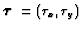wind stress in m2s-2. For the single gyre wind forcing,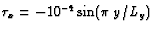and.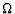basin domain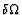boundary of the basin domain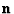normal vector oriented outward the domain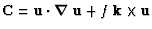Advection-Coriolis termsPrevious: Introduction
Next: The Time Discretization
Up: No Title

Frederic Dupont
2001-09-11